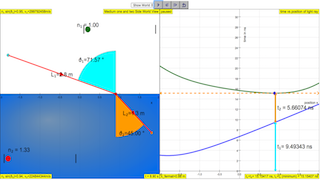## Topics

Light
Refraction of light
Modeling for Snell's Law

## Description

In optics, Fermat's principle or the principle of least time is the principle that the path taken between two points by a ray of light is the path that can be traversed in the least time. This principle is sometimes taken as the definition of a ray of light.
In this Fermat Principle Model, the simulation shows the light path in the least possible time.
Play with the Model. Test what you've learned Snell's Law relationship sin i / sin r = constant and test it's idea against the Fermat's principle.

## Sample Learning Goals

(c) recall and use the terms for refraction, including normal, angle of incidence and angle of refraction
(d) recall and apply the relationship sin i / sin r = constant to new situations or to solve related problems
(e) define refractive index of a medium in terms of the ratio of speed of light in vacuum and in the medium

## Activities

1. Select the (drag and release the dots) desired position 1 and 2 of which light ray (RED) must be transmitted and received.
2. Click run and the simulation will make the point of ray with the medium 1 and 2 interface, move and compute the following assuming point 1 (start TEAL color) and point 2 (end ORANGE color)
1. L1=Math.sqrt((xc-x1)*(xc-x1)+(yc-y1)*(yc-y1))
2. L2=Math.sqrt((xc-x2)*(xc-x2)+(yc-y2)*(yc-y2))
3. time taken in medium 1, t1=n1*L1/c where n1 is refractive index of medium 1, c is speed of light
4. time taken in medium 1, t2=n2*L2/c; where n2 is refractive index of medium
3. using the evidences of t1 + t2 graph of ( time vs position x) , suggests what is the path that has the smallest travelling time, in other words the meaning of t1 + t2 is a minimum?
4. using Snell's Law, verify if Fermat's principle is agreeable to Snell's Law?
5. Calculate the error in the Snell's Law, n₁ sin(ϑ₁)=n₂ sin(ϑ₂) and Fermat's principle.
6. Suggest a reason why the 2 answers are not exactly identical.

## Version:

### Translations

Code Language Translator Run### Software Requirements

SoftwareRequirements

 Android iOS Windows MacOS with best with Chrome Chrome Chrome Chrome support full-screen? Yes. Chrome/Opera No. Firefox/ Samsung Internet Not yet Yes Yes cannot work on some mobile browser that don't understand JavaScript such as..... cannot work on Internet Explorer 9 and below

### CreditsFu-Kwun Hwang; lookang; tina

### Versions

1. http://weelookang.blogspot.sg/2015/07/ejss-fermats-principle-refraction-model.html JavaScript version of Fermat's principle by Fu-Kwun Hwang and Loo Kang Wee
2. http://www.phy.ntnu.edu.tw/ntnujava/index.php?topic=2494.0 Java version of Fermat's principle by Fu-Kwun Hwang

### Other Resources

Rating 5.00 (1 Vote)